# Math Worksheets for 2nd Graders

📆 26 Sep 2022
🔖 Math Category
📂 Gallery Type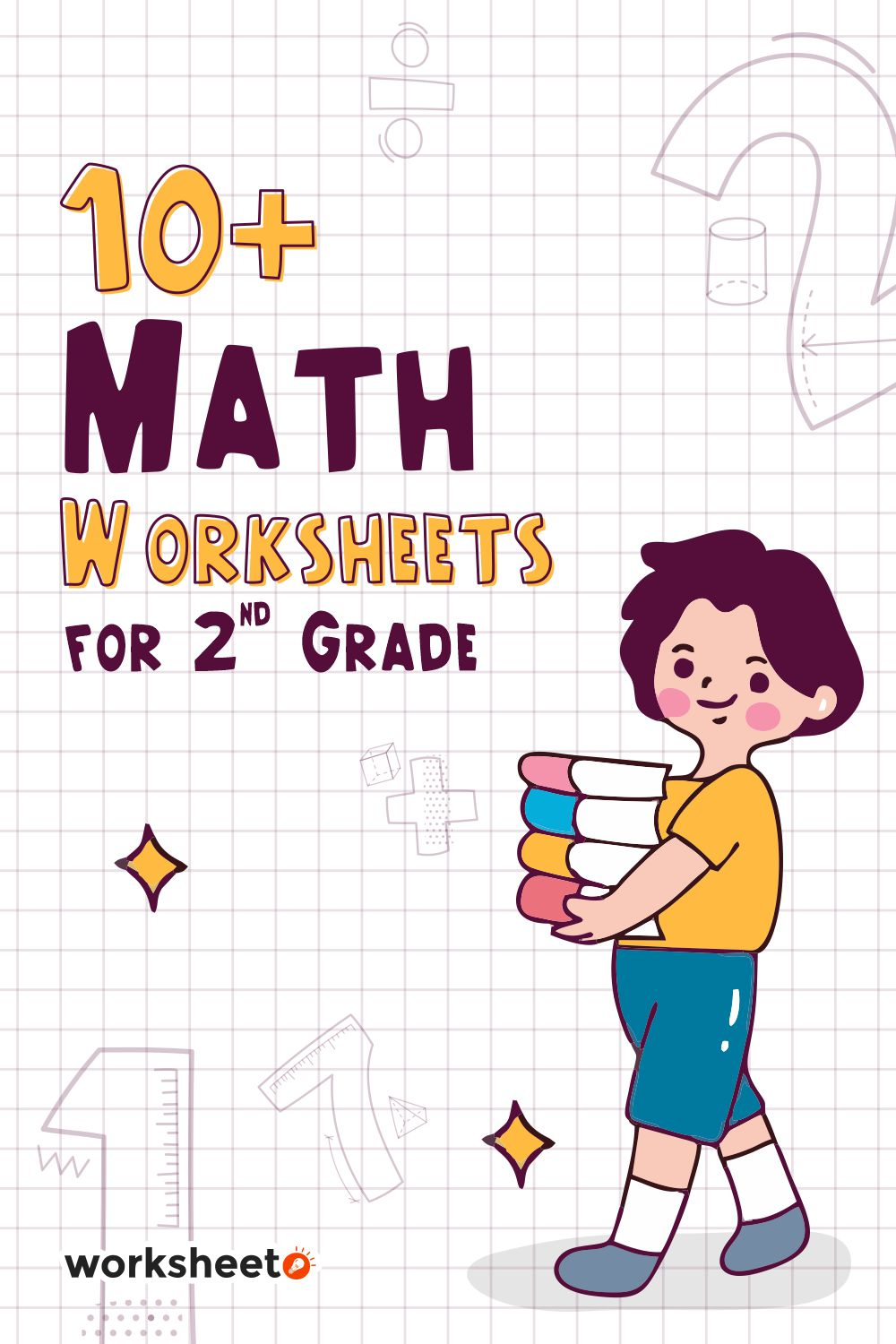14 Images of Math Worksheets For 2nd Graders

Math Worksheets for 2nd Graders are colorful and FUN. They are perfect for practicing math skills with your child. The sheets have different activities and problems to help your child improve their math skills.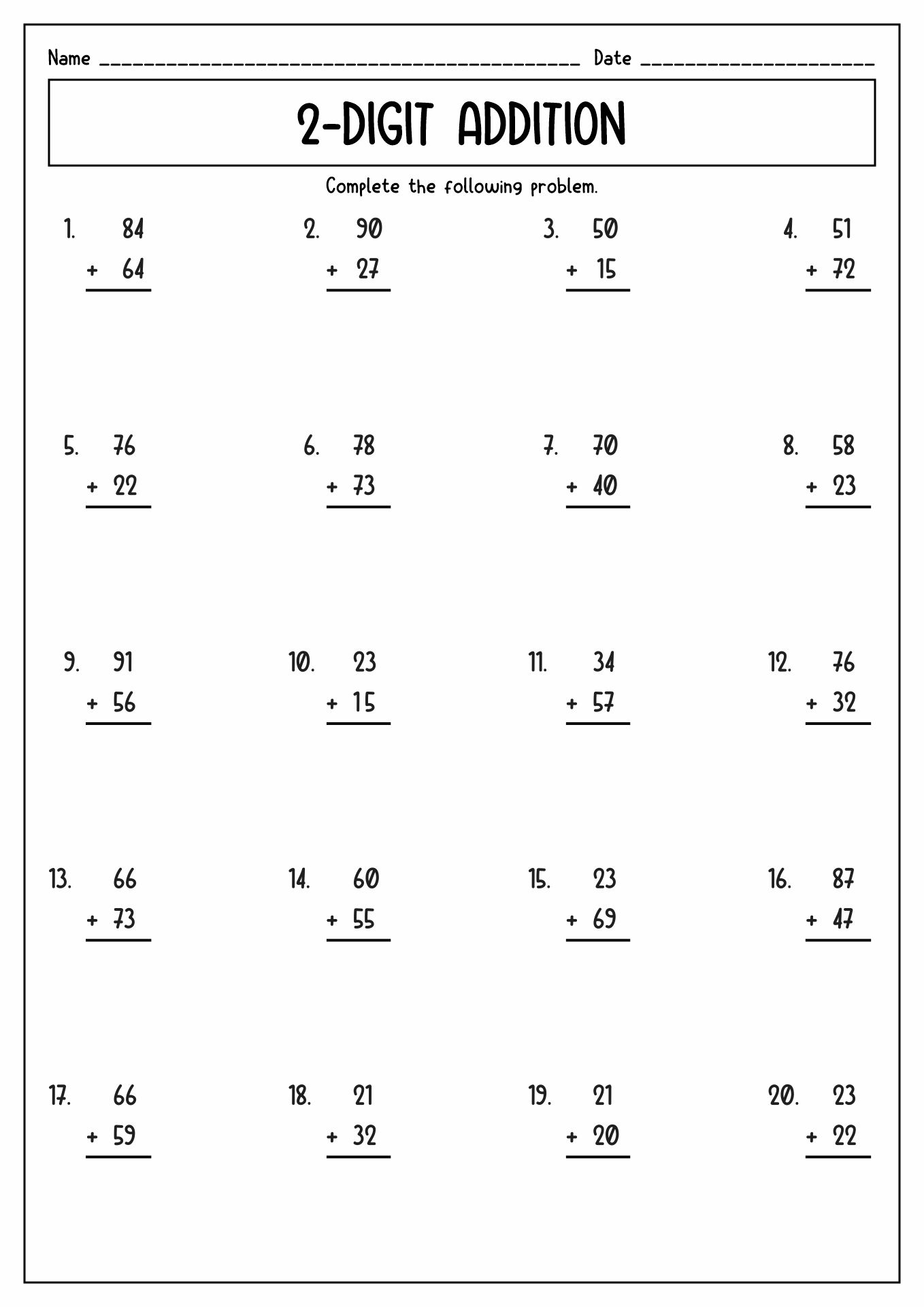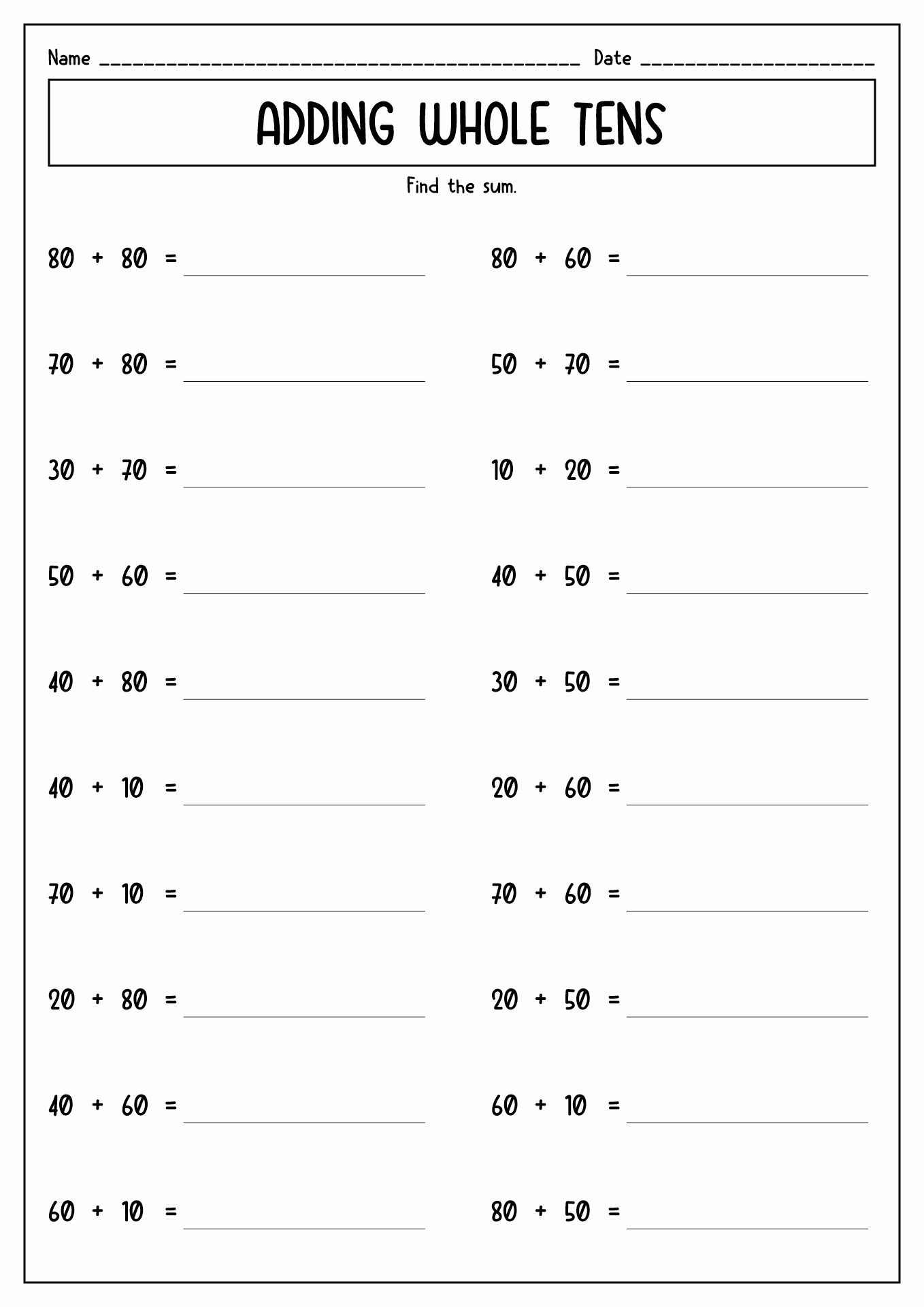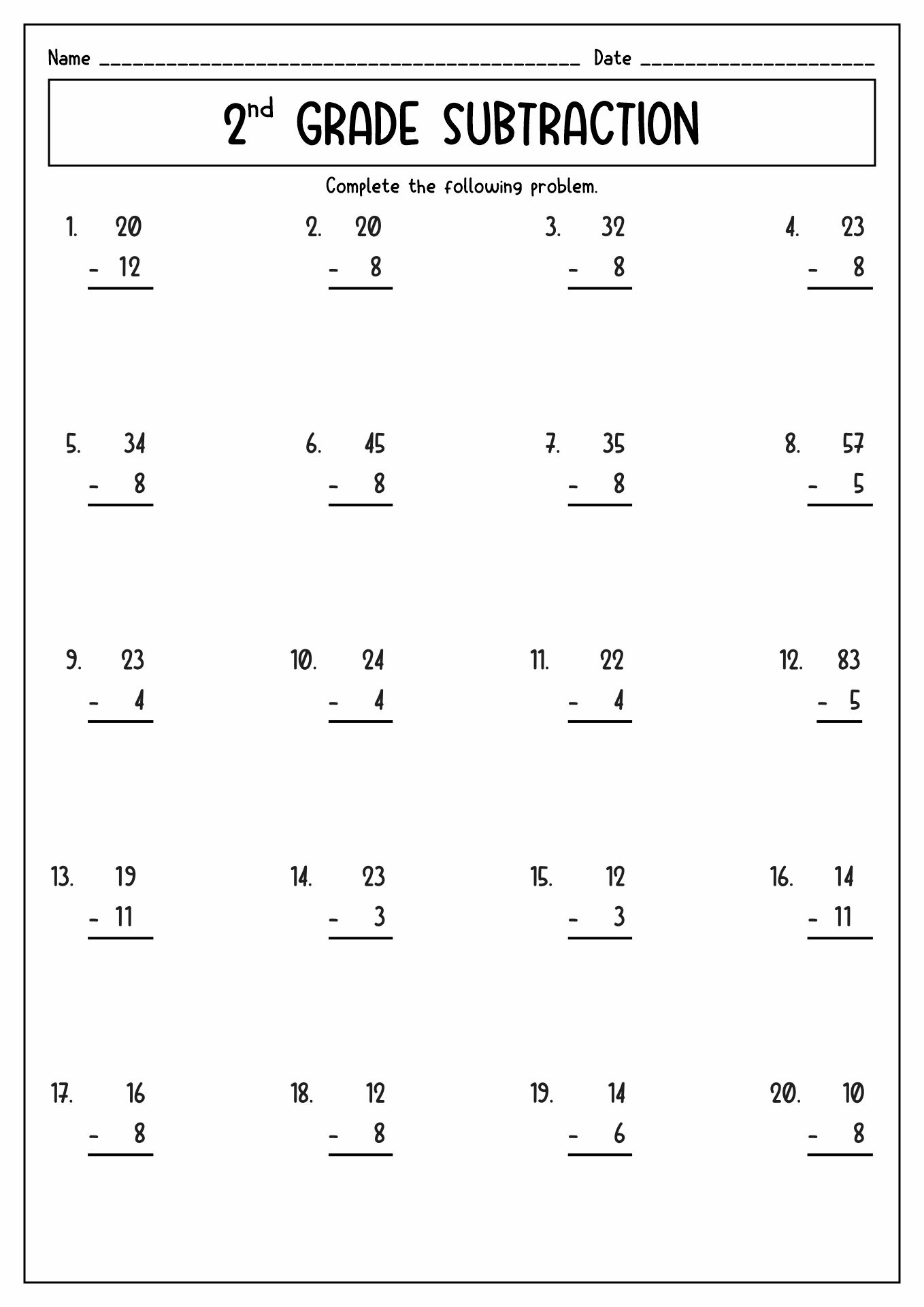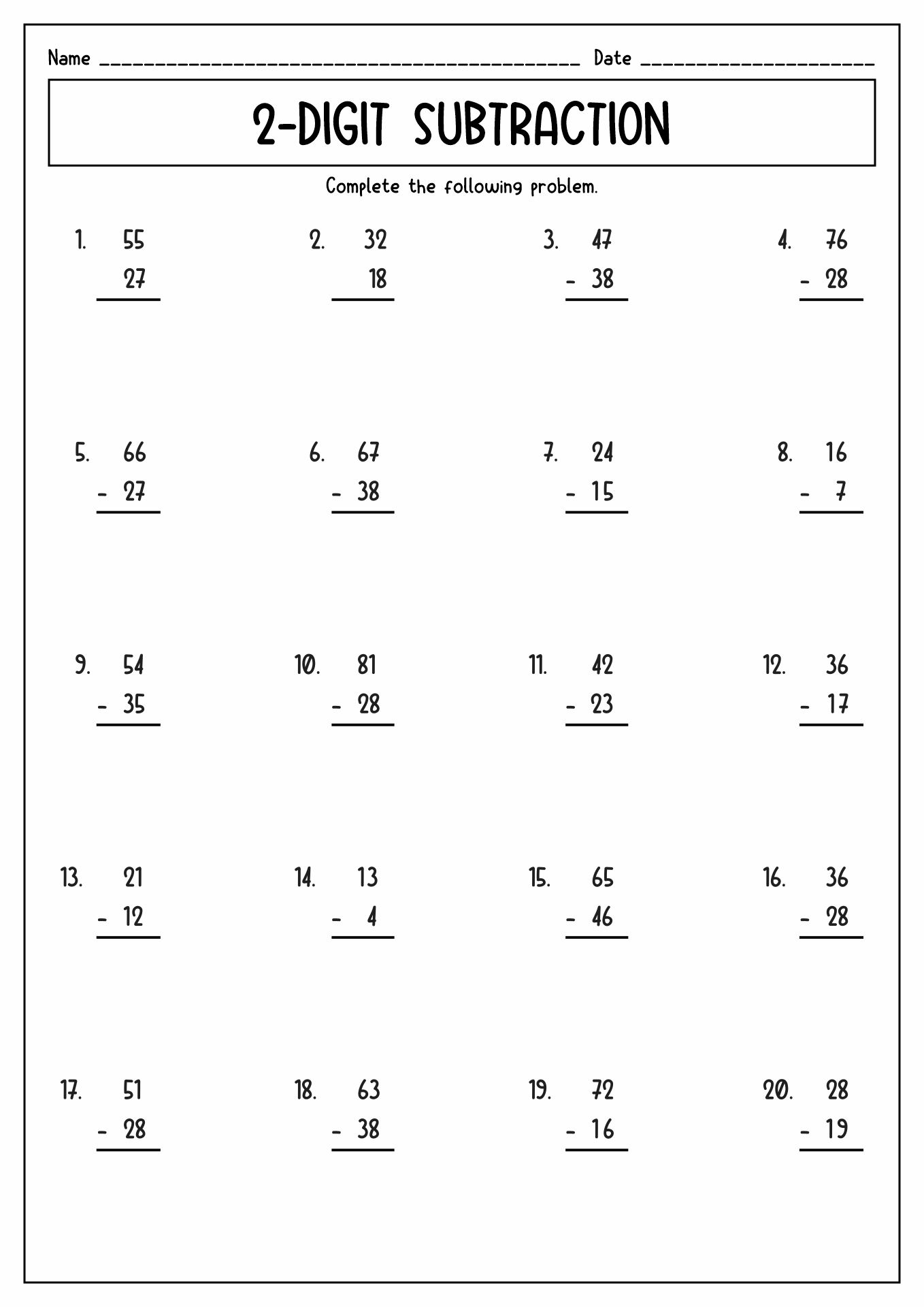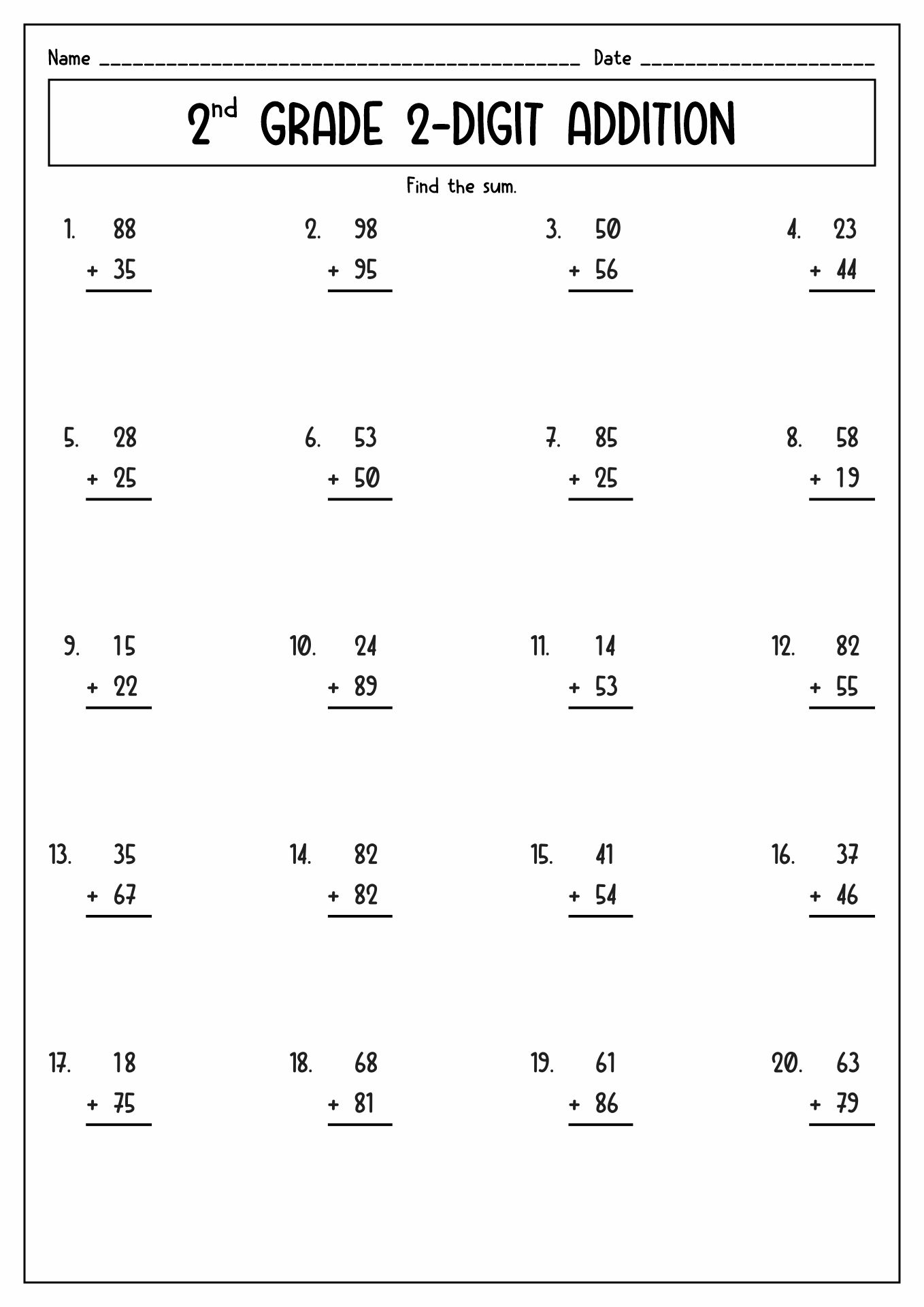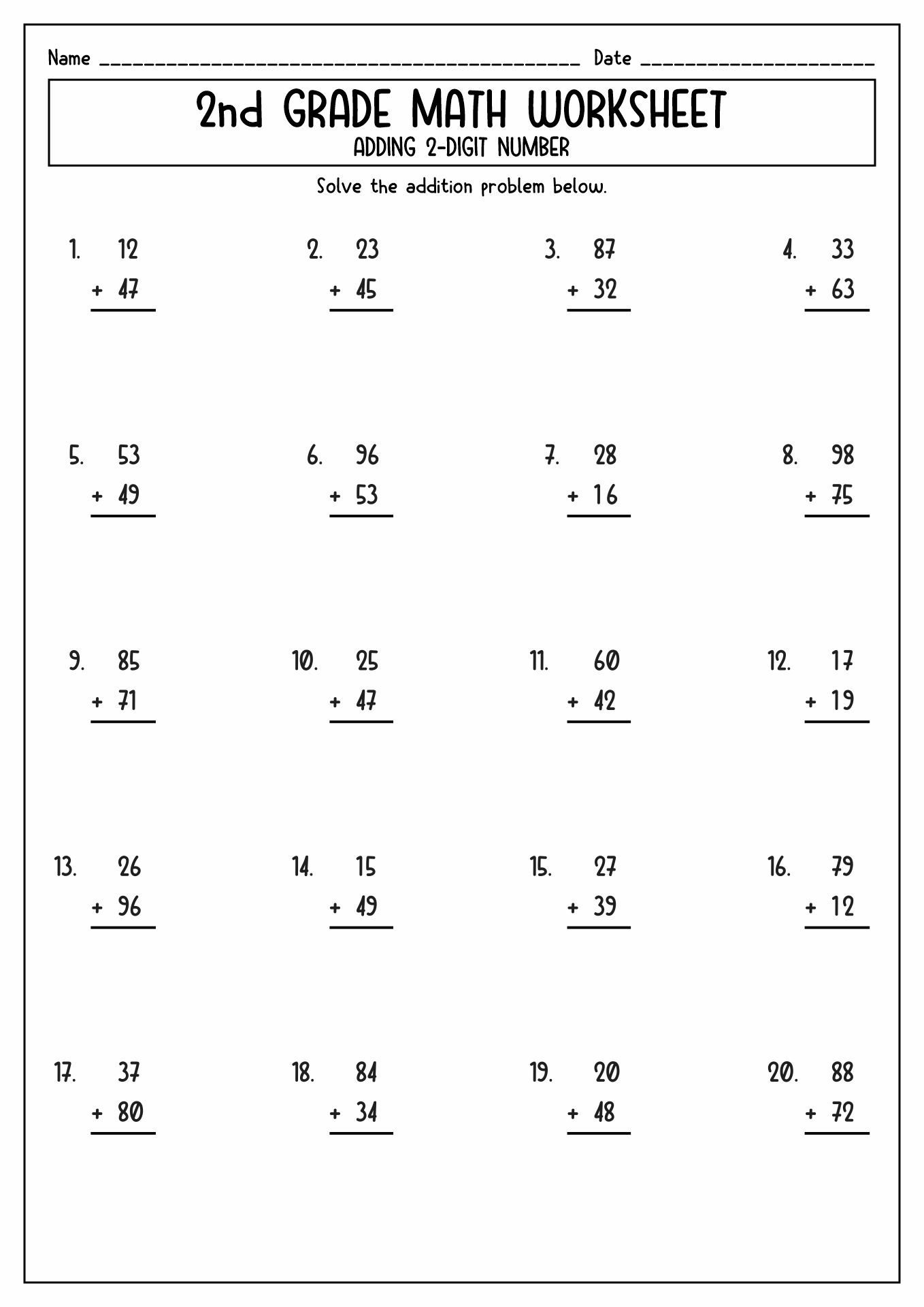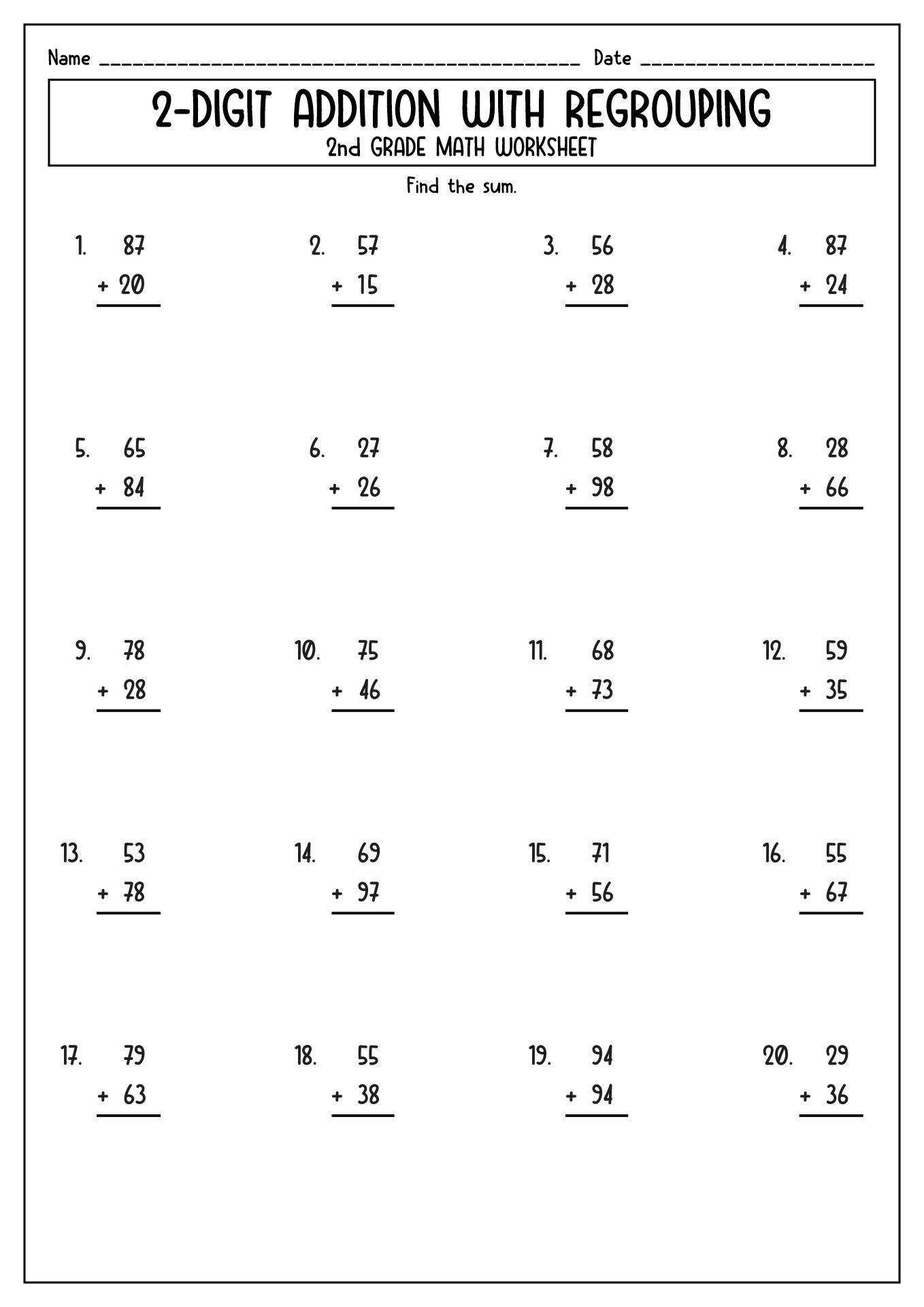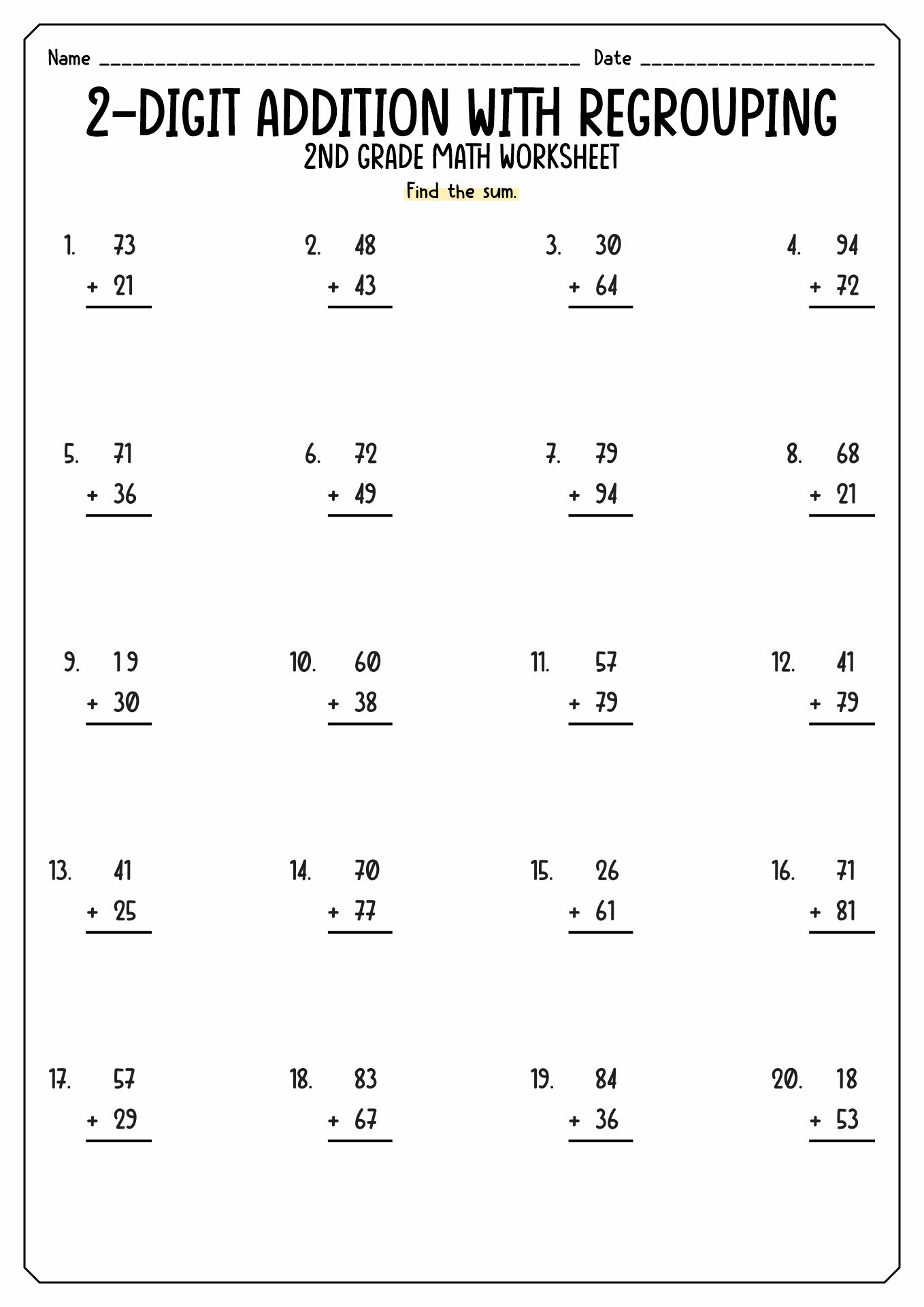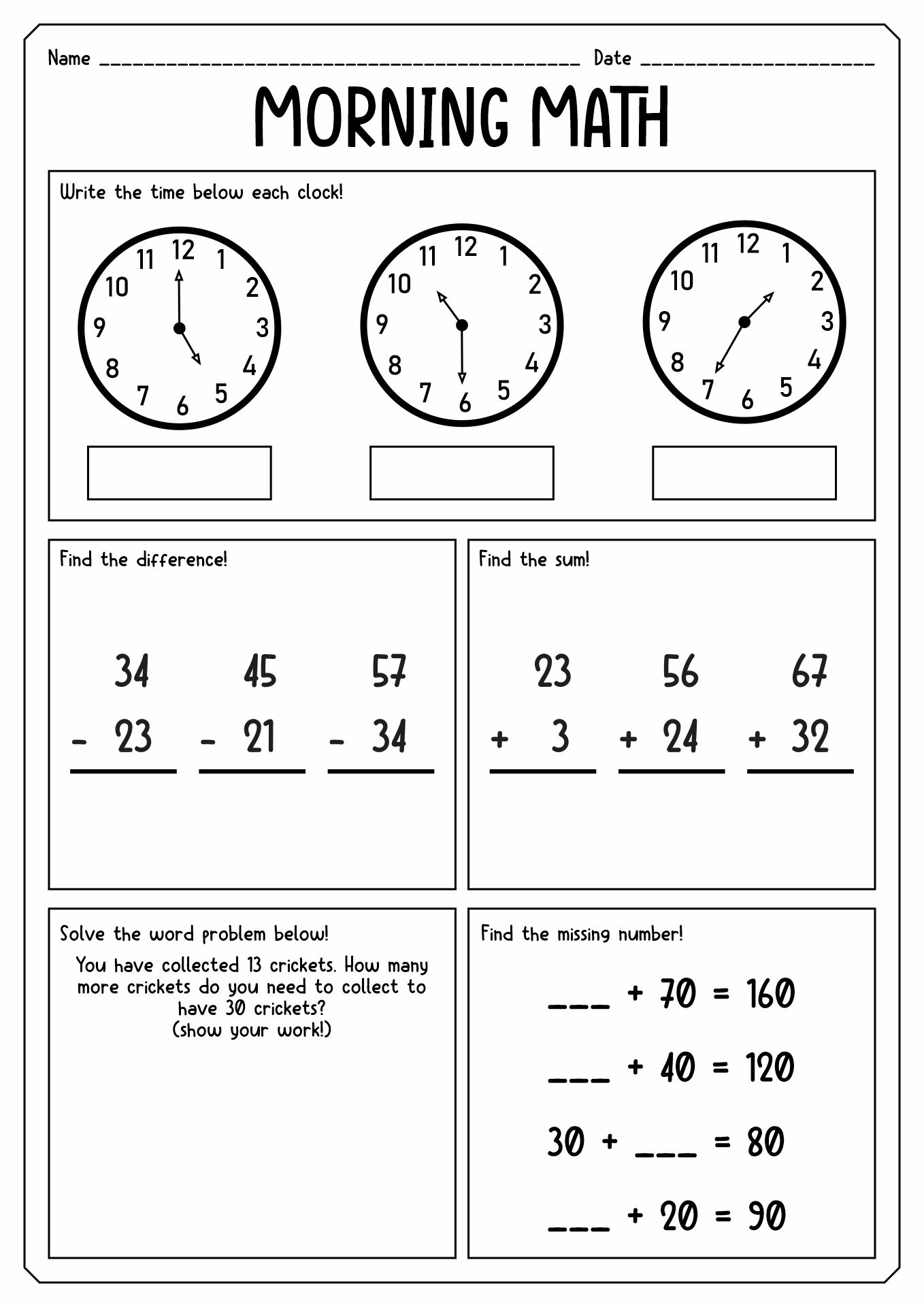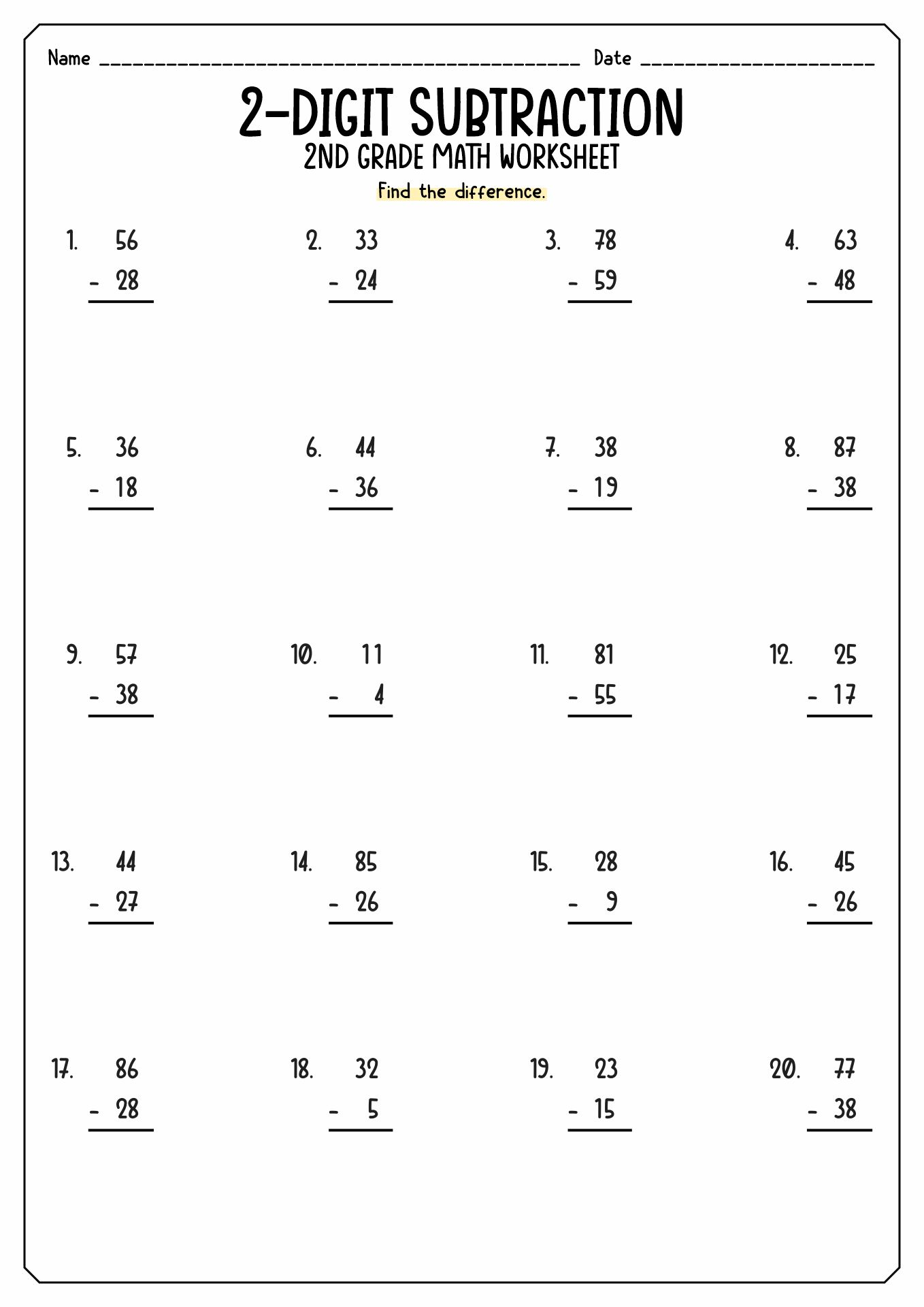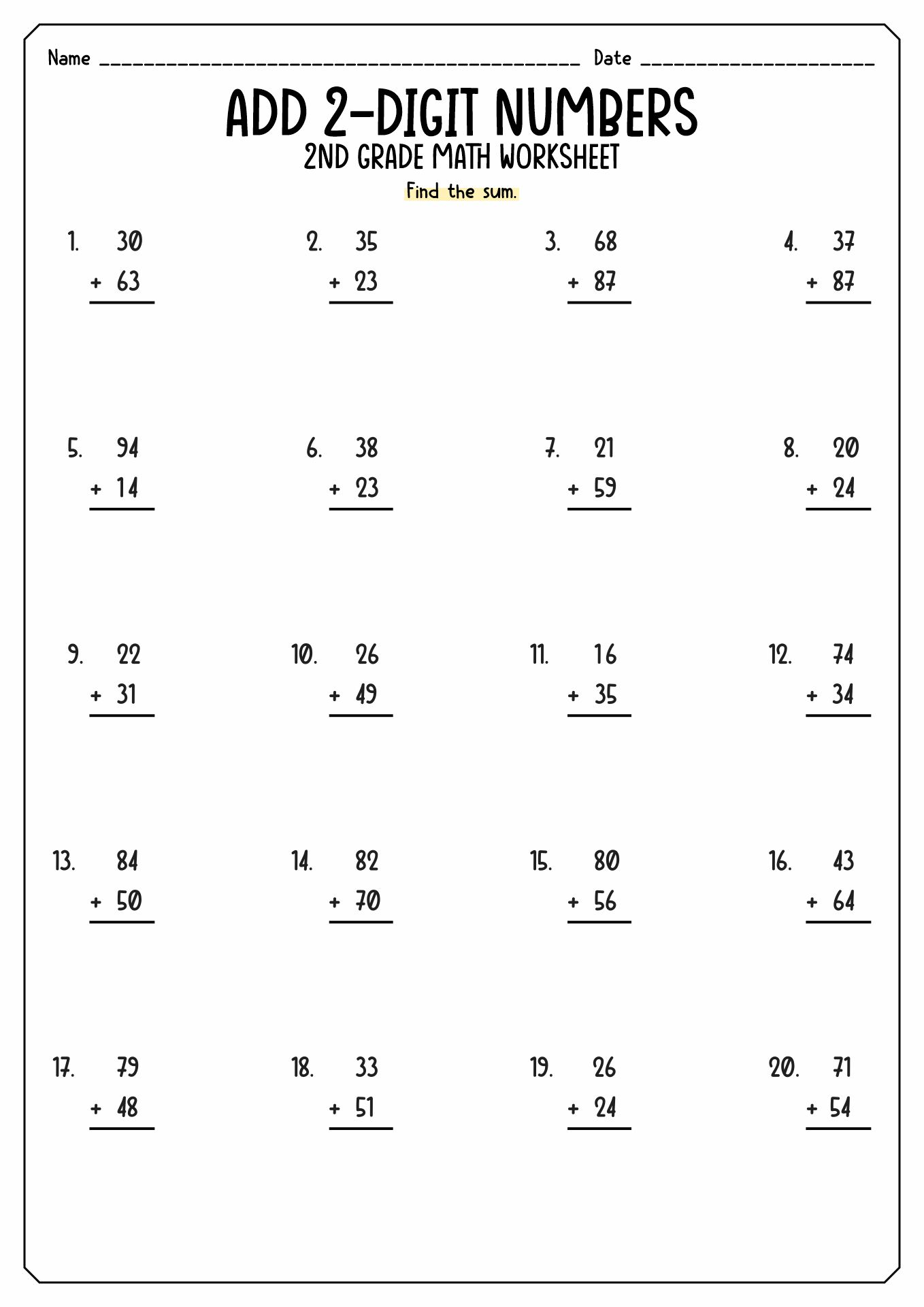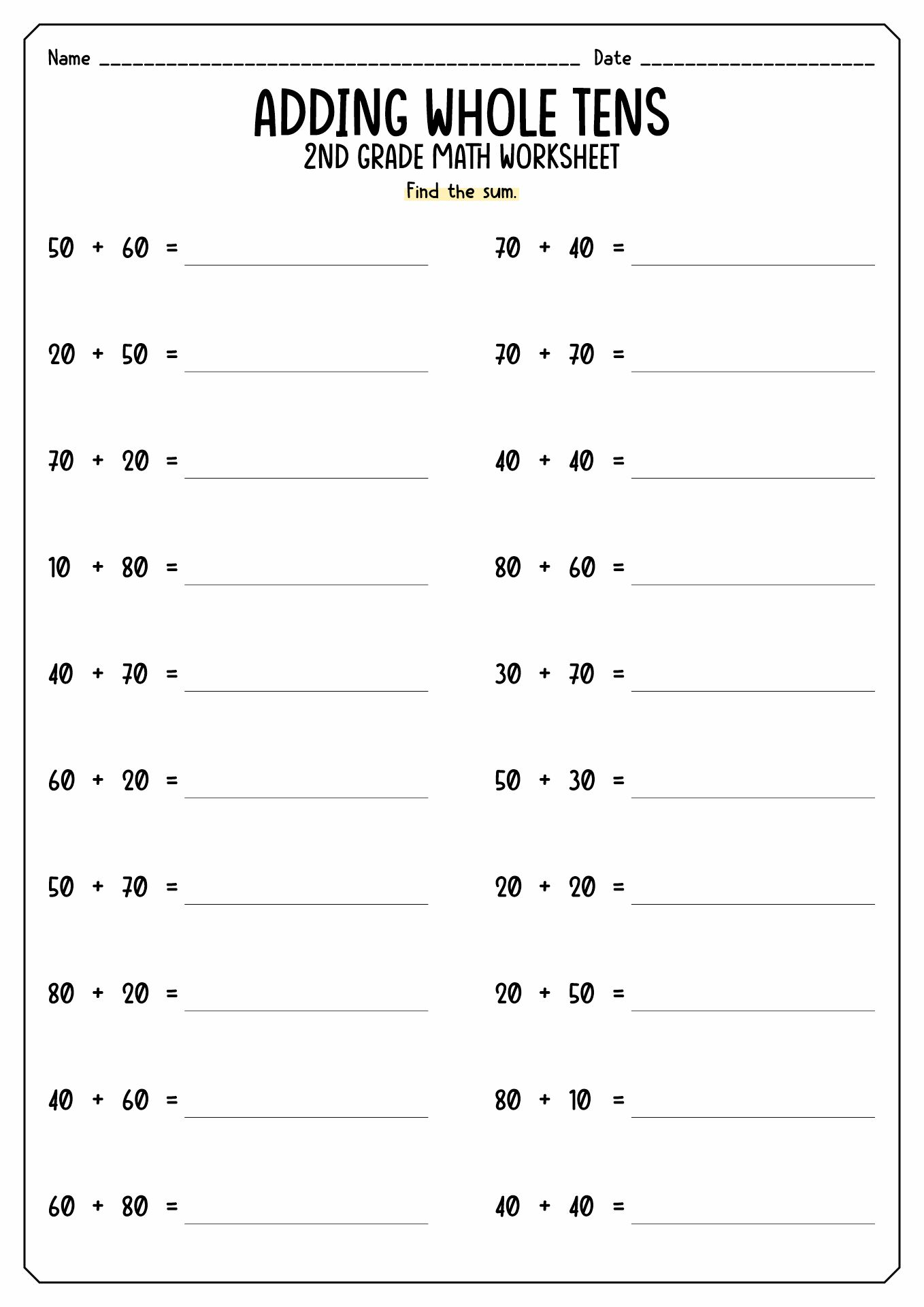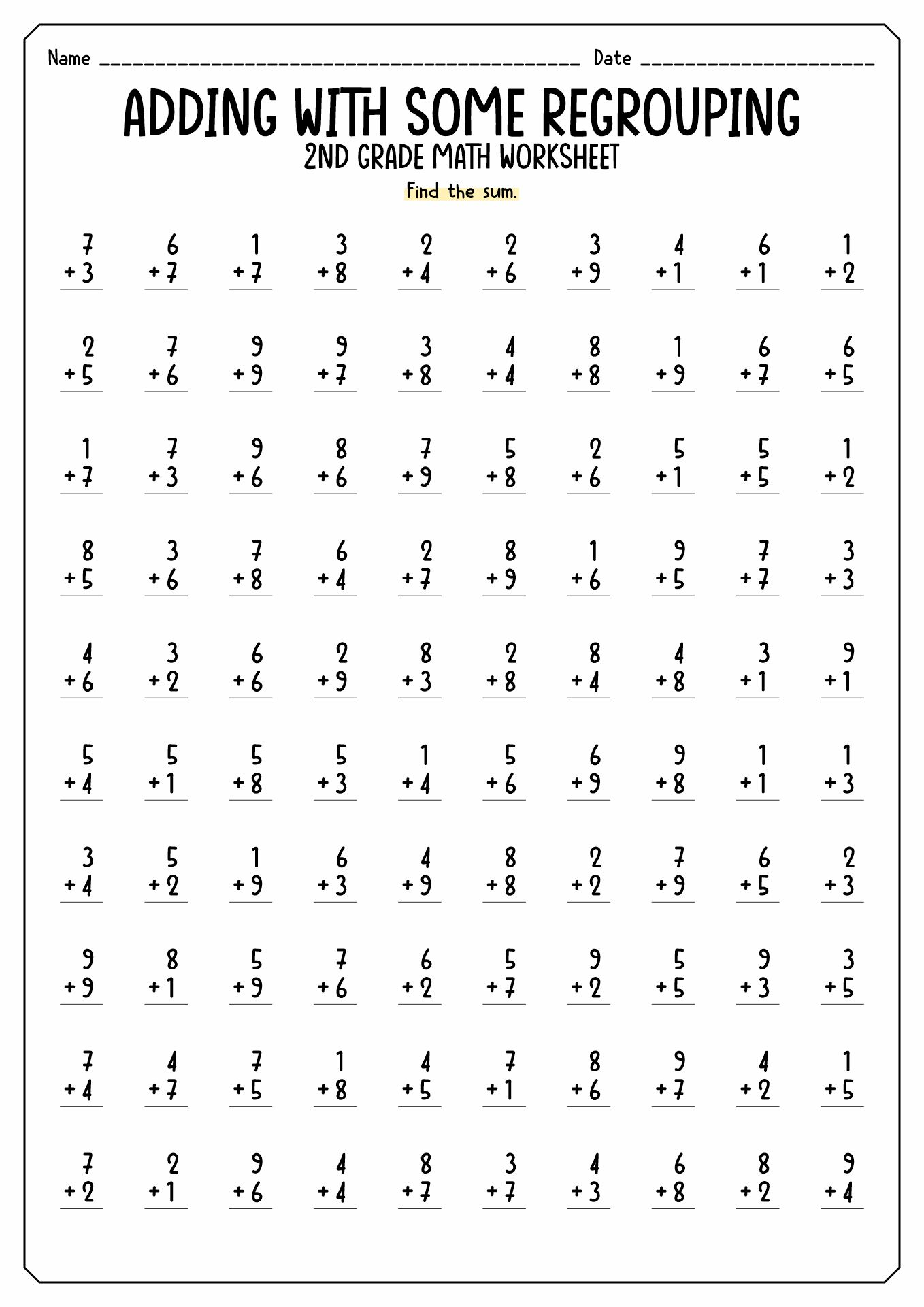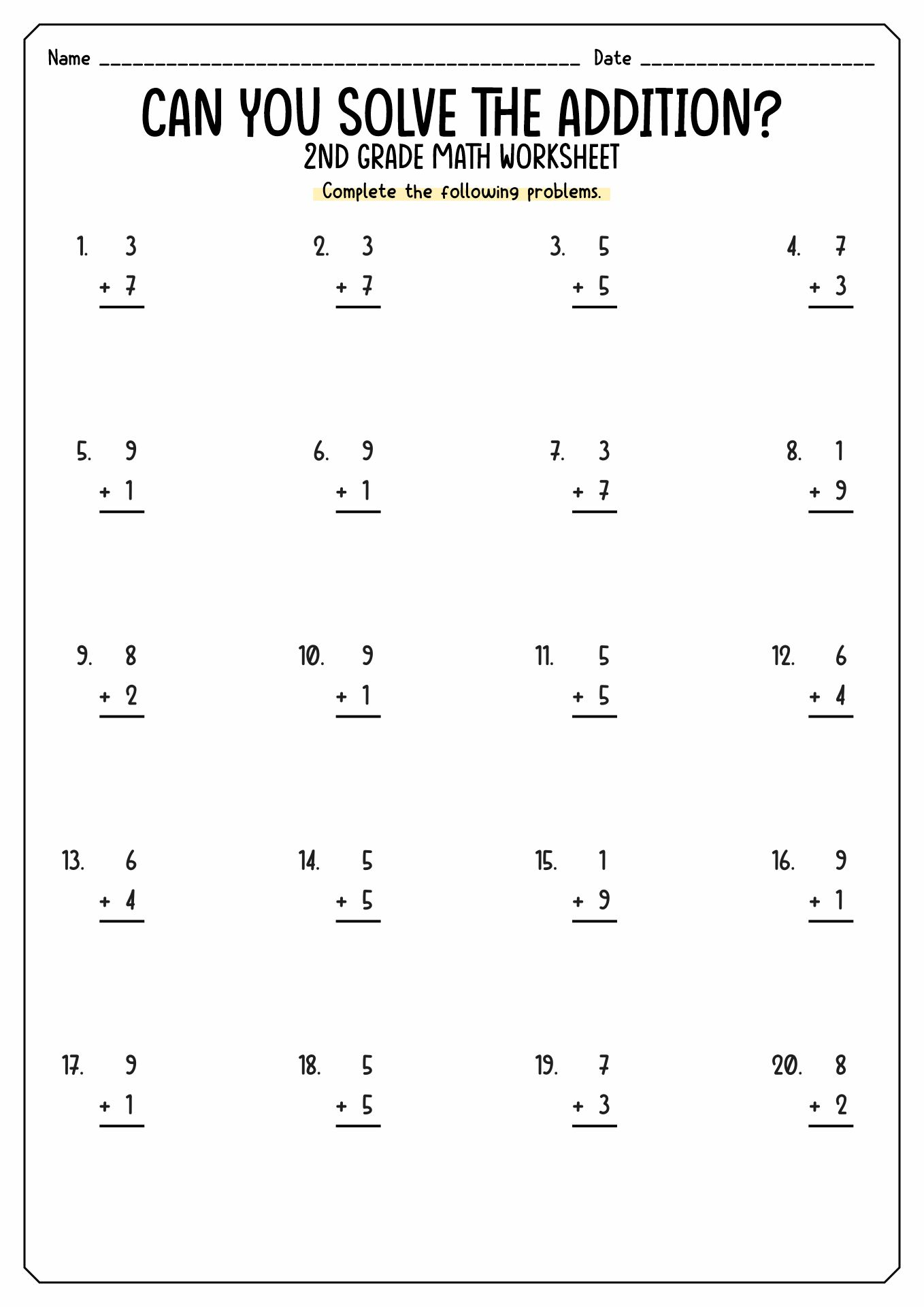Summary: Mathematics is the foundation of many aspects of our surroundings, whether latent or apparent. Hence, mastering mathematic skills from a young age is crucial for children. The early years of elementary school also have a role in solidifying the mathematics role for the children. The ability to count to 1000, addition and subtraction, measurement, and shapes are some mathematics topics that second graders should master.

### What are Early Mathematic Skills for Second Graders?

It is not an overstatement when we say that mathematics is one of the vital science for human's everyday life. It is the foundation of many aspects of our surroundings, whether latent or apparent. Hence, mastering mathematic skills from a young age is crucial for children. Young children can absorb every piece of knowledge they receive as their brain still develops. The preschool stage is crucial to building the base foundation for numerical recognition. However, the early years of elementary school also have a role in solidifying the mathematics role for the children.

Below is some mathematical knowledge to master by second graders, according to the Seattle Public Schools:

• Count and work with numbers up to 1000 digits.
• Able to solve addition and subtraction problems within the range of 100.
• Understand various units of measurement (length, time and money)
• Comprehend the types of shapes (2D and 3D)
• Master the introduction of multiplication.

Addition and subtraction are two of the four arithmetic operations children should master in their early education years. Addition means to combine or add two or more numbers. Meanwhile, subtraction is taking a number or amount from another. These two operations have a tight bond which makes the students should master them back-to-back. These are the foundation for young learners to solve more complex operations such as multiplication (repeated addition) and division (repeated subtraction). It is a crucial skill that can help young students become automatic and fluent in counting. The lesson on addition and subtraction for second graders includes numbers, quantities and measures. The teacher can use various learning mediums such as objects, worksheets, diagrams and symbols ( +, -, and =). Below are some tips for teaching addition and subtraction to second graders from Oxford Owl of Oxford University:

• Train the students to understand and memorize the number facts pairs. Number facts are a pair of addition or subtraction number that we recognize immediately without counting them (4+4 = 8, 5+10 + 15 and more).
• Focus the learning process on subtraction.
• Encourage and help the young learners to keep practice for counting.
• Give tips to the students that subtraction means the differences between the numbers. This tip can make the students understand the operation easier and grasp the concept better.

### Why is Learning Measurement for Second Graders Important?

Second graders students will learn about simple measurement in their mathematics class. This lesson includes the calculations in length, time, and money. At first, the parents or teachers could teach the students to measure something with objects instead of a ruler. For example, check the length of a book with marbles or buttons. Repeat this practice several times until the children grasp the concept. After that, introduce them to the use of a ruler or other tools (depending on what object to measure). Measurement will help us to describe our surroundings in accurate numbers. This lesson will be the foundation for students in their future education and career. Some work requires an accurate measurement for the success of their work, such as engineering, banking, science, accounting and others.

### How to Introduce Shapes to Second Graders?

The shape is an outline of an object. The shape lesson is one of the crucial lessons in athematic as it helps us to recognize our surroundings. The outline or boundary of the object is the combination of curves, points and lines elements. There are two types of shapes, two-dimensional and three-dimensional. Two-dimensional (2D) shapes consist of length and breadth. Meanwhile, Three-dimensional (3D) has three elements (length, width and height). Circles, squares, rectangles, triangles and ovals are some examples of 2D shapes. Meanwhile, cubes, cuboids, cylinders, cones and spheres are examples of 3D shapes. The parents and teachers can introduce the young students to shapes through these steps: introduce what is the definition of shapes, identify the shapes, play and explore the shapes, draw, colour and make crafts with shapes and practice using shapes.

### What are the Fun and Exciting Mathematic Activities for Second Graders?

It is not a secret that mathematics is the enemy of some students. Hence, teachers should hone their creative skills to deliver a fun and exciting learning activity for their classes. It is better to ensure the learning should be fun and still help the students understand the lesson. Below are some fun mathematics activities for second graders:

• Use flashcards to practice addition.
• Practice number facts with a marker spinner.
• Use number search worksheets to practice number facts.
• Play with dice to compare values.
• Do a place-value scavenger hunt.
• Play hopscotch to practice number counting.
• Solve puzzles with skip counting strategy.

The information, names, images and video detail mentioned are the property of their respective owners & source.

### Popular Categories

Have something to tell us about the gallery?

Submit